ET online
March 2016
Current transformers in electrical diagnostics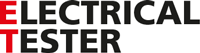# Current transformers in electrical diagnostics

01 March 2016

Dr Stan Zurek, MSc, PhD, SMIEEE - Manager of magnetic development

In the first part of this article, which appeared in an earlier issue of Electrical Tester, we looked at the use of CTs in evaluating the condition of electrical insulation, particular in relation to portable appliance testing (PAT testing). In this second and concluding part, we consider the role of CTs in earth resistance measurement. Note that Figures 1 to 3 appeared with and relate to the first part of the article, which can still be accessed on line.

Earth Resistance
CTs are very useful in the diagnostics of earth or ground resistance. A large building or installation can have multiple earthing points (Fig. 4). Each earthing electrode needs regular inspection.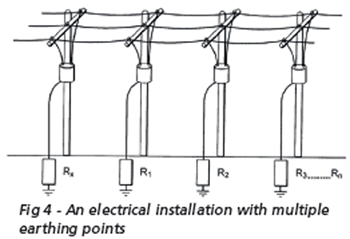The equivalent electric circuit is such that all the earth resistances are connected in parallel. If there are sufficiently many branches, the circuit simplifies to a single resistance in a loop (Fig. 5), and just three branches allow good indication of problematic earth point.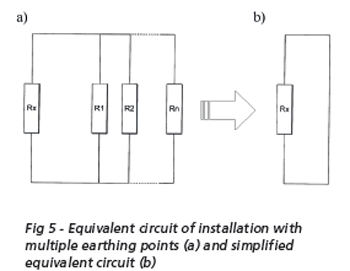For instance, let us assume that a good electrode has a resistance of 1 Ω and a faulty value is 10 Ω. There are two good electrodes and one faulty electrode. Then measurement at a good electrode will give Rx = 1 + 1||10 = 1.9 Ω, but measurement at the faulty electrode will give Rx = 10 + 1||1 = 10.5 Ω. Therefore, the faulty electrode is easily identified. The values are more accurate with more parallel branches.

The measurement would be very simple (applying Ohm’s law) if it could be carried out directly. However, direct measurement is not possible because this would mean introducing a break in the loop shown in Fig. 5b. This is undesirable for safety reasons, because if a fault occurred in the installation while the loop was broken, large potential could appear and could endanger the operator or any other personnel in the vicinity of the equipment. Moreover, after re-connection there could be still doubt about the quality of connection.

A method is therefore needed where the measurement can be carried out in a noninvasive way. This can be achieved with clamp-on current transformers (Fig. 6). One of the greatest advantages of these devices is that they can be clamped around a conductor without breaking the current path and accurate measurements of AC currents can be made.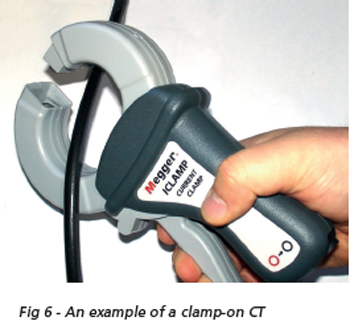Even contactless resistance measurement simplifies to the application of Ohm’s law. Under normal conditions the resistive component of impedance is dominant; capacitive and inductive contributions can be neglected. Therefore, voltage applied to the circuit generates a current that is inversely proportional to the resistance. Measuring AC current with a clampon CT is fairly easy. It requires only the CT and an ammeter connected to its output. The Current transformers in electrical diagnostics difficulty lies in the application of a known voltage to a continuous circuit. However, this can be achieved by using a voltage transformer (VT).

A CT without anything connected to its output is in fact a step-up VT. So if the secondary winding of such a transformer is driven with AC voltage, then the device becomes a step-down VT.

The CT shown in Fig. 6 has a ratio of 1000:1 so an AC current of 1 A in the primary cable produces 1 mA of the output current. But the same device can be used as a VT, and if driven from the secondary side the ratio is still 1000:1, so 1 V applied to the secondary winding will induce 1 mV in the primary cable. Combining VT and CT allows the measurement of resistance value in the loop. The principle is shown in Fig. 7 and a practical instrument in Fig. 8.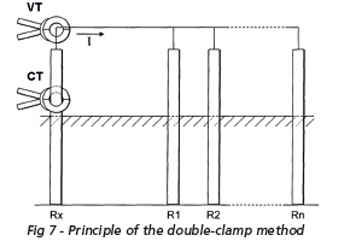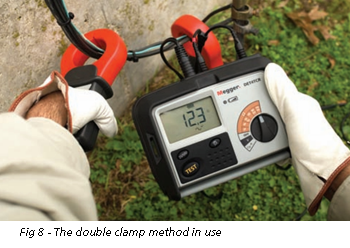For this particular instrument the voltage applied is 30 V at 128 Hz. If the loop resistance is 1 Ω, this will generate a loop current of 30 mA, which will be stepped down to 30 μA by the CT. The test instrument can measure the voltage applied to the VT and the output current of the CT, and the ratios of the VT and CT are constant. Hence, in this case the loop resistance can be calculated as:

Rx = VVT ÷ (ICT ∙1000∙1000) Ω

where: VVT = voltage applied by the instrument to the VT and ICT = output current from the CT as measured by the instrument.

It is also possible to integrate both transformers (VT and CT) into a single device (Fig 9). This instrument operates on the same principle as that discussed above, with two small differences: a higher operating frequency is used because the crosssection area of the cores is smaller, and there is an additional magnetic shielding between the internal VT and CT to improve measurement of small currents in the proximity of the energised VT core.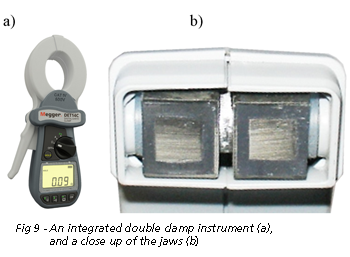The resistance range is from 0.05 Ω to 1500 Ω. Thus, the CT has to be able to detect currents from less than 20 μA to 1 A, because the induced loop voltage is at the level of 30 mV. Such measurement must be performed in the presence of much greater leakage currents, which might be present due to the connection to the 50 Hz (or 60 Hz) network.

Summary
Current transformers are used for precise measurements relevant to safety and reliability in electrical installations.

Two examples were discussed in this series of articles. The first shows the use of current transformers in portable appliance testing where the leakage current in non-isolated devices needs to be detected differentially at the level of 0.0001% or 1 ppm (10 μA in 10 A). Only current transformers are capable of performing such measurement with sufficient accuracy without using very expensive instrumentation or components.

The other application is a contactless measurement of earth resistance with multiple grounding electrodes. A current transformer in normal mode is used for current detection. Voltage is injected by driving a current transformer from its secondary side so that it behaves like a voltage transformer. The loop resistance can be thus calculated from the known voltage and measured current. The two transformers (voltage and current) can be integrated into a single instrument, to produce an easy-to-use handheld device.

In essence, the versatility and performance of current transformers mean that many of the instruments used for testing and diagnostics of electrical insulation and earthing can be made smaller, lighter, safer, more reliable and less expensive.

To see how CTs are used in the earth test range click here